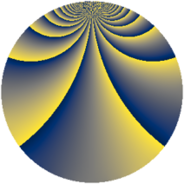# Properties

 Label 2205.2.cqLevel $2205$ Weight $2$ Character orbit 2205.cq Rep. character $\chi_{2205}(121,\cdot)$ Character field $\Q(\zeta_{21})$ Dimension $2688$ Sturm bound $672$

# Related objects

## Defining parameters

 Level: $$N$$ $$=$$ $$2205 = 3^{2} \cdot 5 \cdot 7^{2}$$ Weight: $$k$$ $$=$$ $$2$$ Character orbit: $$[\chi]$$ $$=$$ 2205.cq (of order $$21$$ and degree $$12$$) Character conductor: $$\operatorname{cond}(\chi)$$ $$=$$ $$441$$ Character field: $$\Q(\zeta_{21})$$ Sturm bound: $$672$$

## Dimensions

The following table gives the dimensions of various subspaces of $$M_{2}(2205, [\chi])$$.

Total New Old
Modular forms 4080 2688 1392
Cusp forms 3984 2688 1296
Eisenstein series 96 0 96

## Trace form

 $$2688q - 448q^{4} + 4q^{5} - 10q^{6} + 2q^{7} - 10q^{9} + O(q^{10})$$ $$2688q - 448q^{4} + 4q^{5} - 10q^{6} + 2q^{7} - 10q^{9} + 130q^{12} - 2q^{13} + 58q^{14} - 448q^{16} + 16q^{17} + 6q^{18} + 4q^{19} + 12q^{20} + 22q^{21} - 78q^{23} + 32q^{24} + 224q^{25} + 8q^{26} + 78q^{27} + 8q^{28} - 78q^{29} - 2q^{30} + 16q^{31} + 40q^{32} + 28q^{33} + 10q^{36} + 26q^{37} + 44q^{38} - 12q^{39} - 10q^{41} - 96q^{42} - 8q^{43} + 22q^{44} - 6q^{45} - 78q^{46} - 20q^{47} + 64q^{48} - 4q^{49} - 26q^{51} + 104q^{52} + 8q^{53} + 30q^{54} - 238q^{56} - 10q^{57} - 20q^{59} - 70q^{60} - 82q^{61} + 24q^{62} - 44q^{63} - 448q^{64} + 190q^{66} + 28q^{67} - 278q^{68} - 144q^{69} + 12q^{70} - 32q^{71} + 6q^{72} + 28q^{73} - 16q^{74} + 16q^{76} - 70q^{77} + 244q^{78} + 4q^{79} - 168q^{80} - 110q^{81} + 68q^{83} + 332q^{84} + 14q^{86} - 138q^{87} + 14q^{89} - 18q^{90} + 22q^{91} - 236q^{92} - 30q^{93} + 24q^{94} - 206q^{96} - 2q^{97} - 102q^{98} - 58q^{99} + O(q^{100})$$

## Decomposition of $$S_{2}^{\mathrm{new}}(2205, [\chi])$$ into newform subspaces

The newforms in this space have not yet been added to the LMFDB.

## Decomposition of $$S_{2}^{\mathrm{old}}(2205, [\chi])$$ into lower level spaces

$$S_{2}^{\mathrm{old}}(2205, [\chi]) \cong$$ $$S_{2}^{\mathrm{new}}(441, [\chi])$$$$^{\oplus 2}$$